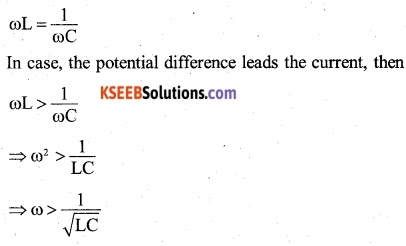# 2nd PUC Physics Question Bank Chapter 6 Electromagnetic Induction

## Karnataka 2nd PUC Physics Question Bank Chapter 6 Electromagnetic Induction

### 2nd PUC Physics Electromagnetic Induction NCERT Text Book Questions and Answers

Question 1.
Predict the direction of induced current in the situations described by the following Figs. 6.18(a) to (f).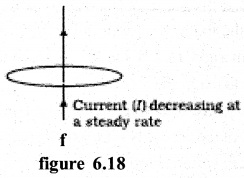(a) According to Lenz’s law south pole forms at q and north pole a to p. Therefore, the induced current is from p to q.
(b) South pole develops at end q and at end x of the two coils as per Lenz’s law. Therefore, the induced current is from q to p in coil PQ and x to y in the coil.
(c) The induced current should flow clockwise in the right loop (when seen from the left) to oppose the current in the left loop. Hence, induced current flows along yzx.
(d) When the rheostat is adjusted to increase the current, magnetic flux through the neighbouring coil increases. As per Lenz’s law, the induced current in the neighbouring coil should produce magnetic flux in opposite direction to oppose the original magnetic flux. Hence, the induced current flows along
(e) When the circuit breaks, magnetic flux decreases. The induced current should flow along xzy to increase the magnetic flux.
(f) The magnetic flux threading the coil in the perpendicular direction is zero. Any change in current will not change this magnetic flux. Hence, no induced current is set up in the coil.Question 2.
Use Lenz’s law to determine the direction of induced current in the situations described by Fig. 6.19:
(a) A wire of irregular shape turning into a circular shape;
(b) A circular loop being deformed into a narrow straight wire.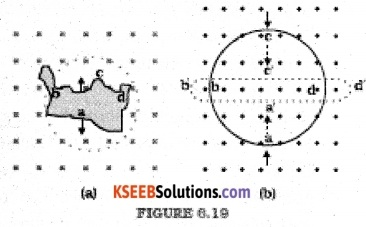(a) The flux linked with the loop increases in this case so flux produced by the induced current should decrease it i.e. the direction of induced current should produce a magnetic field in a direction outwards the plane of the paper. The direction of current as per the right-hand thumb rule comes out to be along adcba. (i.e. anti­clockwise)

(b) In this case, the flux linked with the loop is decreasing so the direction of the induced current is anticlockwise so as to oppose the decrease in flux. Thus, the direction of the induced current is along a’d’c’b’.

Question 3.
A long solenoid with 15 turns per cm has a small loop of area 2.0 cm2 placed inside the solenoid normal to its axis. If the current carried by the solenoid changes steadily from 2.0 A to 4.0 A in 0.1 s, what is the induced emf in the loop while the current is changing?
The magnetic field produced inside the solenoid,
B = µ0 nl
If A is the area of the loop placed inside the solenoid, then magnetic flux linked with the loop,
Φ = BA = µ0 n IA
If e is the induced e.m.f. produced due to change in current through the solenoid, then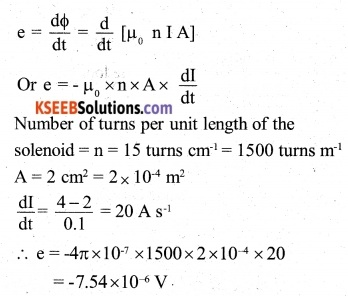Question 4.
A rectangular wire loop of sides 8 cm and 2 cm with a small cut is moving, out of a region of the uniform magnetic field of magnitude 0.3 T directed normal to the loop. What is the emf developed across the cut if the velocity of the loop is 1 cm s-1 in a direction normal to the (a) longer side, (b) shorter side of the loop? For how long does the induced voltage last in each case?Here, B=0.3 T,V=1 cm S-1 10-2 ms-1
In the above expression, 1 is the length of the arm, which is perpendicular to the direction of motion.
(a) B = 0.3 T, l = 8 x 10-2 m, v = 1 x 10-2 ms-1
$$\mathscr{E}$$ = Blv = 0.3 x 10-2 x 8 x 10-2 = 0.24 mV
v = $$\frac { l }{ t }$$
∴ t = $$\frac { l }{ v }$$
= $$\frac { 2 cm }{ 1 cm/s }$$
= 8 sec

(b) The emf developed when the velocity of loop is in the direction normal to the shorter side
$$\mathscr{E}$$ = 0.3 x 10-2 x 2 x 10-2 = 0.06 mV
t = $$\frac { l }{ v }$$
= $$\frac { 8 cm }{ 1 cm/s }$$
= 8 sec

Question 5.
A 1.0 m long metallic rod is rotated with an angular frequency of 400 rad s-1 about an axis normal to the rod passing through its one end. The other end of the rod is in contact with a circular metallic ring. A constant and uniform magnetic field of 0.5 T parallel to the axis exists everywhere. Calculate the emf developed between the centre and the ring.
The induced e.m.f. between the center and the circumference of the ring is given by
e = $$\frac { 1 }{ 2 }$$ Bl2 ω[in magnitude]
Here, B = 0.5 T; 1 = tn; ω=400 rad s-1
∴e= $$\frac { 1 }{ 2 }$$ x 0.5 x l2 x 400 = 100V

Question 6.
A circular coil of radius 8.0 cm and 20 turns is rotated about its vertical diameter with an angular speed of 50 rad s_1 in a uniform horizontal magnetic field of magnitude
3.0 x 10-2 Obtain the maximum and average emf induced in the coil. If the coil forms a closed loop of resistance 10 q, calculate the maximum value of current in the coil. Calculate the average power loss due to Joule heating. Where does this power come from?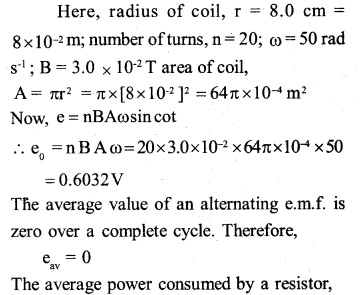The external agent, which keeps the coil rotating, is the source of this power.Question 7.
A horizontal straight wire 10 m long extending from east to west is falling with a speed of 5.0 m s-1, at right angles to the horizontal component of the earth’s magnetic field, 0.30 × 104 Wbm2.
(a) What is the instantaneous value of the emf induced in the wire?
(b) What is the direction of the emf?
(c) Which end of the wire is at the higher electrical potential?
(a) $$\mathscr{E}$$ = Blv = 0.3 x 10-4 x 10 x 5 = 1.5 mv
(b) West to east
(c) Eastern end

Question 8.
Current in a circuit falls from 5.0 A to 0.0 A in 0.1 s. If an average emf of 200 V induced, give an estimate of the self­inductance of the circuit.Question 9.
A pair of adjacent coils has a mutual inductance of 1.5 H. If the current in one coil changes from 0 to 20 A in 0.5 s, what is the change of flux linkage with the other coil?
Using dΦ = Mdl, we get
dΦ= 1.5 X (20 – 0) = 30 Wb

Question 10.
A jet plane is traveling towards the west at a speed of 1800 km/h. What is the voltage difference developed between the ends of the wing having a span of 25 m, if the Earth’s magnetic field at the location has a magnitude of 5 x 10-4 T and the dip angle is 30°?
$$\mathscr{E}$$ = Bv lv = (B sin 30)lv
= 5 x 10-4 x $$\frac { 1 }{ 2 }$$ x 25 x $$\frac { 180×5 }{ 18 }$$
= 3.125 V

### 2nd PUC Physics Electromagnetic Induction Additional Exercises

Question 11.
Suppose the loop in Exercise 6.4 is stationary but the current feeding the electromagnet that produces the magnetic field is gradually reduced so that the field decreases from its initial value of 0.3 T at the rate of 0.02 Ts-1. If the cut is joined and the loop has a resistance of 1.6 q, how much power is dissipated by the loop as heat? What is the source of this power?
Here, area of the loop,Question 12
A square loop of side 12 cm with its sides parallel to X and Y axes is moved with a velocity of 8 cm s-1 in the positive x-direction in an environment containing a magnetic field in the positive Z-direction. The field is neither uniform in space nor constant in time. It has a gradient of 10-3 T cm-1 along the negative x-direction (that is it increases by 103 T cm-1 as one move in the negative x-direction), and it is decreasing in time at the rate of 10 3 Ts-1. Determine the direction and magnitude of the induced current in the loop if its resistance is 4.50 mΩ.
Here, area of the loop,The induced e.m.f produced due to spatial variation of the magnetic field.According to Lenz’s law, the induced current flows through the loop in a direction so as to cause an increase in the magnetic flux along the positive Z-direction. To an observer, the current will appear to be flowing in an anti-clock-wise direction, if the loop is moving towards the right.

Question 13.
It is desired to measure the magnitude of the field between the poles of a powerful loudspeaker magnet. A small flat search coil of area 2 cm2 with 25 closely wound turns, is positioned normal to the field direction, and then quickly snatched out of the field region. Equivalently, one can give it a quick 90° turn to bring its plane parallel to the field direction). The total charge flew in the coil (measured by a ballistic galvanometer connected to the coil) is 7.5 mC. The combined resistance of the coil and the galvanometer is 0.50 Q. Estimate the field strength of the magnet.
Here, number of turns, N = 25
area of coil, A= 2.0 cm2 =2.0 x 10-4 m2
charge flown in the coil, q = 7.5 m
C =7.5 × 10-3 C; resistance of coil R= 0.50 mΩ.
Let B be the strength of the magnetic field, then, magnetic flux linked with the search coil initially.
φ= BAN
When the search coil is brought out of the magnetic field, the magnetic flux linked with the coil finally,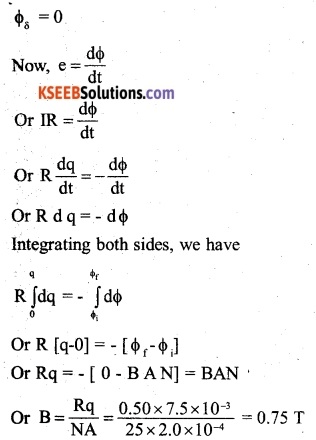Question 14.
Figure 6.20 shows a metal rod PQ resting on the smooth rails AB and positioned between the poles of a permanent magnet. The rails, the rod, and the magnetic field are in three mutually perpendicular directions. A galvanometer G connects the rails through a switch K. Length of the rod = 15 cm, B = 0.50 T, resistance of the closed-loop containing the rod = 9.0 Assume the field to be uniform.(a) Suppose K is open and the rod is moved with a speed of 12 cm s_1 in the direction shown. Give the polarity and magnitude of the induced emf.

(b) Is there an excess charge built up at the ends of the rods when K is open? What if K is closed?

(c) With K open and the rod moving uniformly, there is no net force on the electrons in the rod PQ even though they do experience a magnetic force due to the motion of the rod. Explain.

(d) What is the retarding force on the rod when K is closed?

(e) How much power is required (by an external agent) to keep the rod moving at the same speed (=12 cm s-1) when K is closed? How much power is required when K is open?

(f) How much power is dissipated as heat in the closed-circuit? What is the source of this power?

(g) What is the induced emf in the moving rod if the magnetic field is parallel to the rails instead of being perpendicular?
(a) Using e = Bυl, we get
e = 0.5 x 12 x 10.2 x 15 x 102
= 9 x 10-3 V
The electrons in the rod will experience force along PQ, so end P becomes positive and Q becomes negative.

(b) On closing the key K, the number of electrons becomes more at end Q. Therefore, excess charge is maintained by the continuous current.

(c) Magnetic force gets cancelled by electric force due to excess charges of opposite sign at the ends of the rod.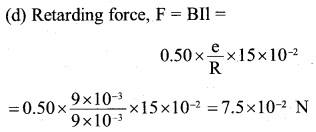(e) When the switch K is closed, the power required by the external agent against the retarding force.
P = Fv = 7.5×10-2×12×10-2 = 9×10-3W

(f) Power dissipated as heat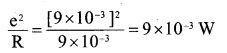The source of this power is the power of external agent against the retarding force,
P = Fv = 7.5×10-2 ×12×10-2= 9×10-3 W

(g) The motion of rod does not cut field lines, hence no induced e.m.f. is produced.Question 15.
An air-cored solenoid with a length of 30 cm, area of cross-section 25 cm2, and a number of turns 500, carries a current of 2.5 A, The current is suddenly switched off in a brief time of 10-3. How much is the average back emf induced across the ends of the open switch in the circuit? Ignore the variation in the magnetic field near the ends of the solenoid.
Here, area of cross-section of the solenoid,Question 16.
(a) Obtain an expression for the mutual inductance between a long straight wire and a square loop of side an as shown in Fig. 6.21.
(b) Now assume that the straight wire carries a current of 50 A and the loop is moved to the right with a constant velocity, v = 10 m/s. Calculate the induced emf in the loop at the instant when x = 0.2 m. Take a = 0.1 m and assume that the loop has a large resistance.(a) Consider as an elementary portion of the area of the loop in the form of a strip parallel to the straight wire having a width dx and at a distance x from the wire as shown in the figure.If dA is are of this strip, then dA = a dx
The magnetic field at the strip due to current-carrying wire,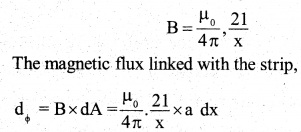The magnetic flux linked with the whole loop can be found by integrating the above between the limits x = r to x = r + b
i.e.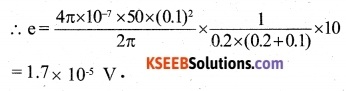Question 17.
A line charge X per unit length is lodged uniformly onto the rim of a wheel of mass M and radius The wheel has light non­conducting spokes and is free to rotate without friction about its axis (Fig. 6.22). A uniform magnetic field extends over a circular region within the rim. It is given by, B = – B0 k (r < a; a < R)= 0 (otherwise).
What is the angular velocity of the wheel after the field is suddenly switched off?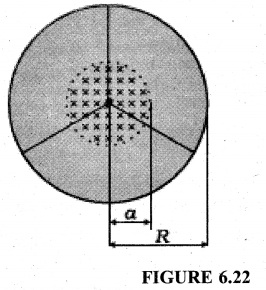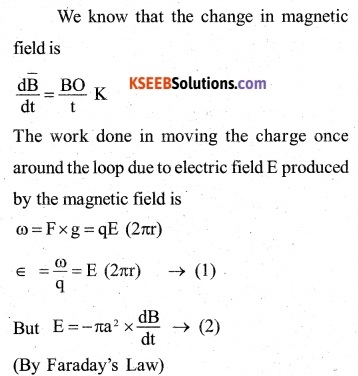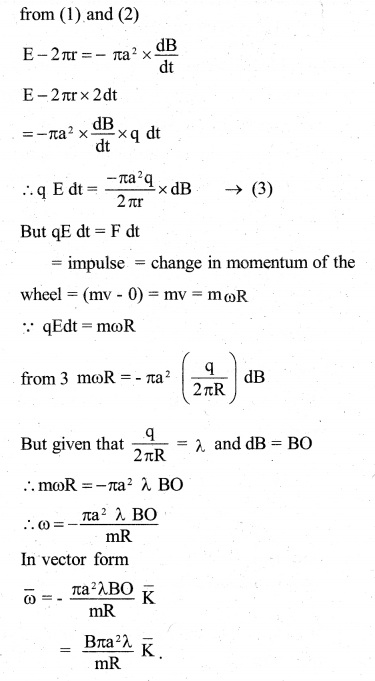Question 1.
Two identical loops, one of copper and another of aluminium are rotated at the same speed in the same magnetic field. In which case, the
(a) induced emf and
(b) induced current will be more and why? (CBSE 2010)
The induced emf will be the same in both but the induced current will be more in the copper loop as its resistance will be lesser as compared to that of aluminium loop.

Question 2.
A plot of magnetic flux (φ) versus current (I) is shown in the figure, for two conductors A and B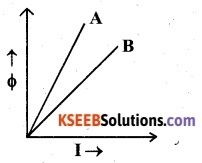Which of the two has a larger value of self-inductance?
From the relation,
φ = LI
it follows that the self-inductance of a conductor is equal to the slope of its graph between φ and I. Therefore, the self-inductance of conductor A has a larger value.

Question 3.
A metal conductor of length lm rotates vertically about one of its ends at angular velocity 5 rad s-1. If the horizontal component of the earth’s magnetic field is 0.2 x 10-4T, then emf developed between the two ends of the conductor is
(A) 5μV
(B) 50μV
(C) 5mV
(D) 50 mV (AIEEE 2004)
The induced emf produced across the two ends of the conductor
e= $$\frac { 1 }{ 2 }$$ Bl2 W=$$\frac { 1 }{ 2 }$$×0.2×10-4×l2 ×5
= 5 ×10-5V = 50 µVQuestion 4.
The self-inductance of the motor of a fan is 10H. In order to impart maximum power at 50Hz, it should be connected to a capacitance of
(A) 4µF
(B) 8µF
(C) 1µF
(D) 2µF (AIEEE 2005)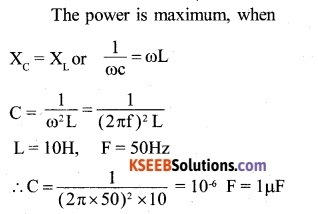Question 5.
Two coils are placed closed to each other. The mutual inductance of the pair of coil depends upon:
(A) The rates at which currents are changing in the two coils
(B) Relative position and orientation of the two coils
(C) The material of wires of the coils
(D) the currents in the two coils
(B) Relative position and orientation of the two coils

Question 6.
A 100 mH coil carries a current of 1A. Energy stored in the form of magnetic field
(A) 0.5J
(B) 1J
(C) 0.05J
(D) 0.1J
L = 100 mH = 0.1. H 1=1 A
The energy stored is given by
U = $$\frac { 1 }{ 2 }$$LI2 = $$\frac { 1 }{ 2 }$$ x 0.1 x l2 = 0.05 J 2

Question 7.
A straight line conductor of length 0.4 m is moved with a speed of 7ms1 perpendicular to the magnetic field of intensity 0.9 Wb nr2. The induced emf across the conductor is.
(A) 1.26 V
(B) 2.52 V
(C) 5.24 V
(D) 25.2 V
B = 0.9 Wb m2
v = 7 ms-1
l = 0.4m
The induced emf produced
φ = BVl = 0.9x 7×0.4
= 2.52 V

Question 8.
What is the power factor of the LCR circuit at resonance?
At resonance, the impedance of the circuit is equal to the resistance in the circuit,
i.e. Z = R
∴ power factor of the circuit,
cos φ= $$\frac { R }{ Z } =\frac { R }{ R } =1$$

Question 9.
In a resonant circuit, at which angular frequency, potential difference leads the current?